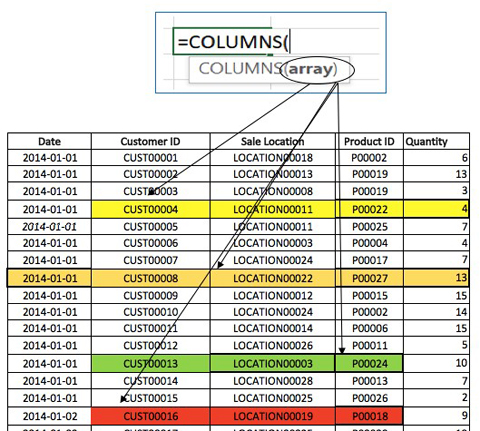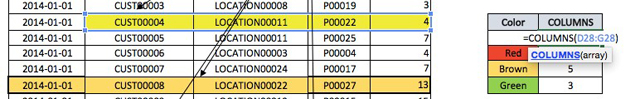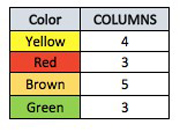COLUMNS FORMULA in Excel
Tuesday, Jul 14# How to Use the COLUMNS FORMULA in Excel

The columns formula in Excel is used to count the number of columns that are selected in a range. The formula is very simple, just select the array or the table which contains the columns that you want to count within the brackets of the columns formula.

Formula explanation:

Array: The cells which are selected to find out the number of columns within the selected area.

Example:

In this example, we are going to find out the number of columns of the selected cells from the following data table.Formula Implementation:Result:This is just a useful formula that comes in handy when you may want to think about using in combination with another formula that you use in your financial modeling depending on the analysis that you’re performing.

I hope that helps. Please leave a comment below with any questions or suggestions. For more in-depth Excel training, checkout our Ultimate Excel Training Course here. Thank you!

Article RatingInline Feedbacks